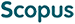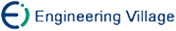Wu Liqiang, Yang Xiaoyuan, Zhang Minqing. Identity-Based Threshold Decryption Scheme from Lattices under the Standard Model[J]. Journal of Computer Research and Development, 2018, 55(10): 2164-2173. doi: 10.7544/issn1000-1239.2018.20180446
 Citation: Wu Liqiang, Yang Xiaoyuan, Zhang Minqing. Identity-Based Threshold Decryption Scheme from Lattices under the Standard Model[J]. Journal of Computer Research and Development, 2018, 55(10): 2164-2173. doi: 10.7544/issn1000-1239.2018.20180446# Identity-Based Threshold Decryption Scheme from Lattices under the Standard Model• The identity-based threshold decryption (IBTD) system combines the secret sharing method with the identity-based encryption mechanism. In a (t, N) IBTD system, N decryption servers share the private key corresponding to a user’s identity. When to decrypt, at least t servers are required to participate in and calculate their corresponding decryption shares. However, less than t or fewer servers are unable to obtain any information about the plaintext. At present, the existing IBTD schemes from lattices are constructed under the random model, and the main method is to divide the private key statistically close to a Gauss distribution directly. This paper constructs a non-interactive IBTD scheme. A public vector is split using the Lagrange secret partition method, and each decryption server obtains its respective characteristic vector. Each private key share is obtained by sampling the pre-image of the characteristic vectors through the private trapdoor function for each decryption server. The user’s complete private key is effectively hidden and the security of the scheme is improved. The difficulty of the discrete logarithm problem is used to realize the verifiability of decryption share. The correctness of the decryption share is guaranteed by the homomorphism of the operations between the common vector and the private key shares. The IND-sID-CPA security for the proposed scheme is proved based on the decisional learning with errors (LWE) hardness assumption under the standard model.
•/DownLoad:  Full-Size Img  PowerPoint